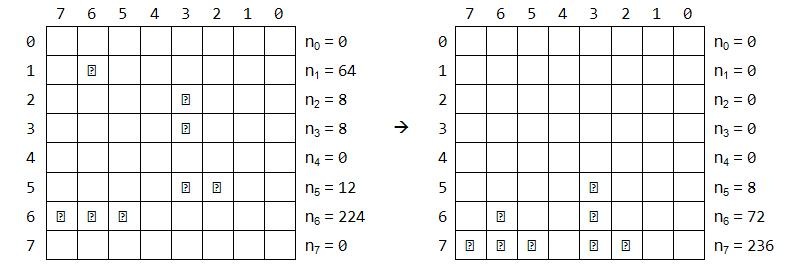# Solving Someone Else’s Bitwise Operator Homework

Here’s an interesting question I came across on Stack Overflow the other day regarding bitwise operations.

The question looks simple enough at first glance, but finding an efficient solution is made difficult by the constraints the author put on it. The question is as follows:

I have the following exercise: The numbers n0 to n7 are bytes represented in binary system. The task is every bit to drop either to the bottom or if it meets another bit it stays above it. Here is a visual example:

I realized that if I apply Bitwise OR on all the numbers from n0 to n7 it’s always the correct result for n7:``````n7 = n0 | n1 | n2 | n3 | n4 | n5 | n6 | n7;
Console.WriteLine(n7); // n7 = 236``````

I looked at this suggestion and noted that n0 was equal to the bit-wise AND on all numbers.

``````n0 = n0 & n1 & n2 & n3 & n4 & n5 & n6 & n7;
Console.WriteLine(n0); // n7 = 0``````

I looked at these two clues and assumed that there was a symmetry to be had across all rows:

``````n7 = n0 | n1 | n2 | n3 | n4 | n5 | n6 | n7;
n6 = n0 | n1 | n2 | n3 | n4 | n5 | n6 & n7;
n5 = n0 | n1 | n2 | n3 | n4 | n5 & n6 & n7;
n4 = n0 | n1 | n2 | n3 | n4 & n5 & n6 & n7;
n3 = n0 | n1 | n2 | n3 & n4 & n5 & n6 & n7;
n2 = n0 | n1 | n2 & n3 & n4 & n5 & n6 & n7;
n1 = n0 | n1 & n2 & n3 & n4 & n5 & n6 & n7;
n0 = n0 & n1 & n2 & n3 & n4 & n5 & n6 & n7;``````

Boom. 25 points here I come. I received an upvote and found myself at + 1.
Then I was at 0.
Then I was at -1.

Confused, I looked over my answer and came to the realization it was completely wrong. If there are two numbers in n0 and n1, the above solution won’t account for them. It assumes the bits have already “fallen” into their correct position, which isn’t the case.

I quickly deleted the answer, embarrassed at my oversight and started thinking about the problem. Essentially, I wanted to count the number of ON bits in a column efficiently. There are a number of tricks to count the number of bits set in a given integer, but none that I knew of to count across integers. I considered adding, subtracting ANDing, ORing, XORing, and just about every operation that one might apply to an integer. Surely there was an efficient way to accomplish what seemed like an incredibly easy problem.

A few answers came in. They involved iterating over the collection multiple times. Some had the advantage of terminating early, but all repetitively churned through the collection multiple times, dropping bits at most one level at a time.

While the answers were all valid, something bugged me about them. It seemed like there had to be a more efficient solution. Something that didn’t need to loop over the whole collection multiple times. I tried to break the problem down. How might we solve it if it was just two numbers?Well, I already knew the answer to this. The top row is simply the AND of both, while the bottom is the OR of both.

``````n0 = n0 & n1;
n1 = n0 | n1;``````If we repeat this on another pair of two, we might get something that looks like:This seems interesting because now we’ve guaranteed that each pair is completely solved. We can take advantage of this in two ways:
1. For each pair, if a topmost bit is set, we know the bottom-most bit is set.
2. For each pair, if a bottom-most bit is not set, the topmost bit is not set.

This allows us to merge the two pairs without checking each possible combination:

``````n0 = (n0_ & n2_);
n1 = (n0_ & n3_) | (n1_ & n2_);
n2 = (n0_ | n2_) | (n1_ | n3_);
n3 = (n1_ | n3_);``````

Essentially, we’ve created a divide and conquer algorithm and taken advantage of correct ordering when we merge the pieces. The rest of the algorithm can be seen here, but it basically just continues the pattern demonstrated above. While it’s not as simple as I had hoped it might be, I was happy to have come up with it.